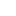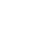# Determination of strength of given strong Acidic solution (hydrochloric acid) by Strong Basic solution (NaOH) by using Conductivity meter

Determination of strength of given strong Acidic solution (hydrochloric acid) by Strong Basic solution (NaOH) by using Conductivity meter

#### Glassware:

Glass ware required for this experiment is listed as:

• A Burette
• 2 Beakers(100&250 ml)
• A Pipette
• A Measuring cylinder
• A Funnel

#### Apparatus:

Following apparatus is required:

• A Conductivity meter
• An Electrical Balance
• Iron Stand
• Clamp

#### Chemicals Required:

The chemicals required are:

• A Sodium Hydroxide Solution (HCl, 31.5-33%)
• A Hydro Chloric Acid Solution (40g/mol)
• Distilled water

#### Theory:

Conductometric is a type of titration in which the electrolytic conductivity of the reaction mixture is continuously monitored as one reactant. The equilance point is that at which the sudden change in conductance is shown by conductivity meter. The point at which the concentration of acidic and basic ions becomes equal is known as equilance point.

#### Conductance:

The reciprocal of resistance is called conductance.
Conductance= 1/Resistance
The units used for conductance are ohm-1 or mho

#### Factors of Conductance:

The conductance of solution is due to:

• Number of ions
• Movements of ions

The reaction in which hydro chloric acidic (HCl) solution is titrated against a solution of sodium hydroxide (NaOH) is given below.
(H+ + Cl-) +Na+ +OH-→ NaCl +H2O

#### Principle:

The addition of an electrolyte to a solution of another electrolyte under the conduction involving non-appreciable change in volume will affect the conductance of the solution whether it is ionic or not.
During titration when a strong acid is added to an alkali solution, the conductance of the reaction will decrease owing to appearance of ions and its replacement with less mobile ions of acid and base.

#### Standard solution:

The solution of known concentration is called standard solution. Here standard is the parameter in which quantity of solute is given i.e. molarity, normality, molality of formality. There are two types of standard solution.

• Primary standard solution
• Secondary standard solution

#### Primary standard solution:

A primary standard solution is that whose concentration remains constant with passage of time is known as Primary standard solution. A primary solution is a reagent that is extremely pure, stable and has no water of hydration for example oxalic acid. Some primary standard solution of titration of acids includes sodium carbonate.

#### Secondary standard solution:

A secondary standard solution is that which is impure, instable, hygroscopic, whose concentration changes with the passage of time. For example sodium hydroxide (NaOH), in open air it absorbs moister and its concentration is changed.

#### Conductivity meter:

An electrical conductivity meter measures the electrical conductivity in a solution in ionic forms. Basically movements of ions produce the conduction. Conductivity meter has two modes.

• Conductance mode
• Resistance mode

#### Procedure:

• First of all, the glassware was washed in order to avoid impurities
• 0.1 M NaOH solution was prepared in 100ml of solution
• 0.1M HCl solution was also prepared in 100ml of solution to use in experiment
• The conductivity meter was calibrated by placing the electrodes in distilled water for five minutes
• HCl solution was filled in burette with the help of funnel as a primary standard
• NaOH solution was taken in titration flask
• Conductivity meter was allowed to set at conductance mode with bright band maxima. The bulb of electrodes was dipped in NaOH solution and titrated against primary solution
• By the addition of HCl solution, change in conductance was monitored. At one point there was sudden change in conductance was examined , volume of standard was noted
• By further addition of HCl, the conductance decreased and a point was reached at which the increase in conductance was noticed. When the increase became constant, titration was stopped
• Calculations were carried out according to readings

#### Observations and Calculations:

Preparation of 0.1M NaOH in 100ml
Amount of NaOH in 1M solution in 1000ml=40g
Amount of NaOH in 1M solution in 1ml=40/1000 g
Amount of NaOH in 1M solution in 100ml=40×100/1000g
Amount of NaOH in 0.1M solution in 100ml=4×0.1g
=0.4g
So, 0.4g of NaOH was weighed on electrical balance and dissolved in distilled water taken in measuring flask. The flask was filled up to the mark.

#### Preparation of 0.1M HCl in 100ml (unknown in experiment)

Percentage purity of HCl=31.5%
Density of HCl=1.16g/cm3
Amount of HCl in 1M solution in 1000ml=36.5g
Amount of HCl in 1M solution in 1ml=36.5/1000 g
Amount of HCl in 1M solution in 100ml=36.5×100/1000g
Amount of HCl in 0.1M solution in 100ml=3.65×0.1g
=0.365g
HCl is always available in solution form, so we have to find volume of HCl.
Volume= Mass/Density
=0.365/1.16
=o.314ml
As solution of HCl is not 100% so further calculations must be carried out
Purity of HCl solution=31.5%
So
31.5ml of HCl are present in =100l
1ml of HCl is present in =100/31.5ml
0.314ml of HCl is present in =100×0.314/51.5ml
=0.99ml=1ml
So 1ml of HCl was taken with the help of measuring cylinder and poured into 100ml measuring flask, and then filled the flask with distilled water up to the mark.
NaOH solution             HCl solution               Conductance
(In mho)
25ml                                       0-2ml                            7.7×10/5
25ml                                       2-4ml                            7.6×10/5
25ml                                       4-6ml                            7.5×10/5
25ml                                       6-8ml                            7.2×10/5
25ml                                       8-12ml                          6.8×10/5
25ml                                      12-16ml                         6.6×10/5
25ml                                      16-20ml                         7.5×10/5
25ml                                      20-22ml                         7.6×10/5
25ml                                      22-26ml                        7.6×10.5

#### Strength of acid (HCl)

Acid      Base
M1V1=M2V2
M2= 0.1m
V2= 25ml
V1= 22ml
M1=?
M1= 0.1×25/22
M1= 0.10m
Strength per liter= Molarity × Molecular mass
= 0.10× 36.5
=3.65 g/L

#### Result:

There is decrease in conductance at first then increase due to decrease in ions first then increase in ions respectively.
The molarity of HCl solution is 0.1m and strength of hydrochloric acid (HCl) solution is 3.65g/L.

#### Precautions:

• Apparatus should be washed carefully and dried properly to avoid impurities
• HCl and NaOH solution should be handled carefully because both in concentrated form are corrosive
• The electrodes of conductivity meter should be handled carefully
• Lab-coat and gloves should be necessary
• Don’t use broken glassware because it can damage and can affect readings
• Take readings carefully and check meniscus of burette properly
• Place the lid of reagent bottles attentially

#### All Practical

Please share All friends Thank You.###### Don’t miss these Chemistry topics!
Sharing Is Caring:

### 3 thoughts on “Determination of strength of given strong Acidic solution (hydrochloric acid) by Strong Basic solution (NaOH) by using Conductivity meter”

1.•2.